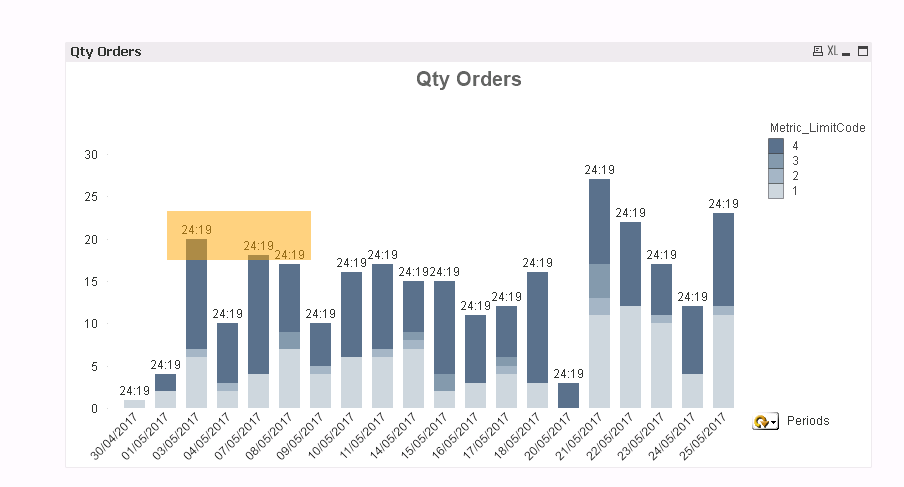# QlikView Layout & Visualizations

Discussion Board for collaboration on QlikView Layout & Visualizations.

Highlighted
Contributor III

## Getcurrentfield in TOTAL expression

Hi,

My bar chart has 2 dimensions:

1.periods - cyclic selection

2. metric code (1-4)

expressions:

1.bar - order quantity

2.text - average duration of all segments for the current period (e.g., date)

I need to display an average for each column, according to a selected cyclic dimension.

But the following expression doesn't work, as all the values are the same.

sum(TOTAL <\$(='[' & GetCurrentField([Cyc_SaleOrd_Periods]) & ']')> Order_OpenTarget_TimeDiff)

/count(TOTAL <\$(='[' & GetCurrentField([Cyc_SaleOrd_Periods]) & ']')>  DISTINCT  SaleOrder_ID)

Would appreciate another way to display it...

Thanks!Message was edited by: Dafnis X Attached sample data

Tags (2)
1 Solution

Accepted Solutions
Valued Contributor

## Re: Getcurrentfield in TOTAL expression

Hello, Dafnis!

It's a great experience to solve your problem. Thank you 😃

There is one more solution which can be the one you lookin' for:

=sum(total <\$(=GetCurrentField(Periods))> Duration)/sum(total <\$(=GetCurrentField(Periods))> [Qty Orders])

We've finnaly reached the GetCurrentField() function =D

12 Replies
Honored Contributor III

## Re: Getcurrentfield in TOTAL expression

Can you share sample data to work with.

Valued Contributor

## Re: Getcurrentfield in TOTAL expression

Hello, Dafnis!

Perhaps, you are getting one value for all columns due to exact a total prefix. Try to use an aggr(sum(),dates)/aggr(count(),dates). I don't sure for real, but in some case it may help.

Contributor III

## Re: Getcurrentfield in TOTAL expression

Hi,

The issue is probably due to the getcurrentfield.. 🙂

Contributor III

## Re: Getcurrentfield in TOTAL expression

Just did..

Thanks!

Honored Contributor III

## Re: Getcurrentfield in TOTAL expression

Try without \$ sign, it may be calculating it to value of the field rather than adding the fieldname to the total.

Contributor III

## Re: Getcurrentfield in TOTAL expression

It gives an error :"allocated memory exceeded"

Valued Contributor

## Re: Getcurrentfield in TOTAL expression

I still don't get why you want to use a total prefix if you want an average for each column in your chart. If I understood you right it should be enough to use expression w/o a total prefix. PFA.

Contributor III

## Re: Getcurrentfield in TOTAL expression

Sergey,

Thanks so much for your kind help!

I probably was wrong with what how I was trying to do it..

For Each bar the value  should display the average duration of an order according to the dimension selected.

If that's what your calculation tries to achieve, that i think it's wrong.

For example:

3/5/2017

sum(duration)/sum(orders) =  1.5 (~30/20)

but you got 2.4

Can you please explain?

Thanks!

Valued Contributor

## Re: Getcurrentfield in TOTAL expression

My apologize, I didn't check result well. 2.4 - is the average of one metric. In case of calculating all metrics using of Total prefix seems to be the way.

So, the solution will be that:

=sum(total <OpenWeek,OpenDate> Duration)/sum(total <OpenWeek,OpenDate> [Qty Orders])